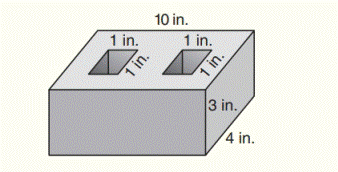Chapter 9.CR, Problem 29CR### Elementary Geometry for College St...

6th Edition
Daniel C. Alexander + 1 other
ISBN: 9781285195698

#### Solutions

Chapter
Section### Elementary Geometry for College St...

6th Edition
Daniel C. Alexander + 1 other
ISBN: 9781285195698
Textbook Problem
1 views

# Find the volume of cement used in the block shows.To determine

To find:

The volume of cement used in the block.

Explanation

Formula used:

Volume of rectangular prism:

V=lwh

Given:

Find the volume of cement used in the block shows.

Calculation:

The cement used in the block is containing a large rectangular prism and two smaller rectangular prisms.

The length of large rectangular prism is l1=10 in.

The width of large rectangular prism is w1=4 in.

The height of large rectangular prism is h1=3 in.

Also,

The length of small rectangular prism is l2=1 in.

The width of small rectangular prism is w2=1 in.

In the figure, the height of the large rectangular prism is equal to the height of the small rectangular prism

The height of small rectangular prism is h2=3 in.

The volume of cement used in the block is,

### Still sussing out bartleby?

Check out a sample textbook solution.

See a sample solution

#### The Solution to Your Study Problems

Bartleby provides explanations to thousands of textbook problems written by our experts, many with advanced degrees!

Get Started

#### Simplify: 728

Elementary Technical Mathematics

#### {x+y+2z=62x+5z=12x+2y+3z=9

Precalculus: Mathematics for Calculus (Standalone Book)

#### Rationalize the denominator of xx42 and simplify.

Mathematical Applications for the Management, Life, and Social Sciences

#### 9. List four mortgage loan closing costs. (14-4)

Contemporary Mathematics for Business & Consumers

#### For y = x sin x, y = _____. a) x cos x b) x cos x + 1 c) cos x d) x cos x + sin x

Study Guide for Stewart's Single Variable Calculus: Early Transcendentals, 8th